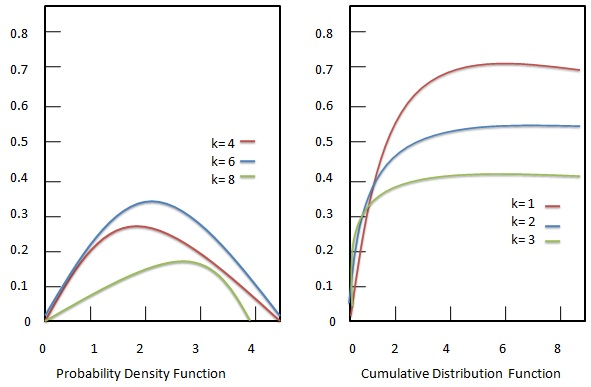# Statistics - Chi-squared Distribution

The chi-squared distribution (chi-square or ${X^2}$ - distribution) with degrees of freedom, k is the distribution of a sum of the squares of k independent standard normal random variables. It is one of the most widely used probability distributions in statistics. It is a special case of the gamma distribution.Chi-squared distribution is widely used by statisticians to compute the following:

• Estimation of Confidence interval for a population standard deviation of a normal distribution using a sample standard deviation.

• To check independence of two criteria of classification of multiple qualitative variables.

• To check the relationships between categorical variables.

• To study the sample variance where the underlying distribution is normal.

• To test deviations of differences between expected and observed frequencies.

• To conduct a The chi-square test (a goodness of fit test).

### Probability density function

Probability density function of Chi-Square distribution is given as:

## Formula

${ f(x; k ) = }$ $\begin {cases} \frac{x^{ \frac{k}{2} - 1} e^{-\frac{x}{2}}}{2^{\frac{k}{2}}\Gamma(\frac{k}{2})}, & \text{if$x \gt 0 $} \\[7pt] 0, & \text{if$x \le 0 $} \end{cases}$

Where −

• ${\Gamma(\frac{k}{2})}$ = Gamma function having closed form values for integer parameter k.

• ${x}$ = random variable.

• ${k}$ = integer parameter.

### Cumulative distribution function

Cumulative distribution function of Chi-Square distribution is given as:

## Formula

${ F(x; k) = \frac{\gamma(\frac{x}{2}, \frac{k}{2})}{\Gamma(\frac{k}{2})}\\[7pt] = P (\frac{x}{2}, \frac{k}{2}) }$

Where −

• ${\gamma(s,t)}$ = lower incomplete gamma function.

• ${P(s,t)}$ = regularized gamma function.

• ${x}$ = random variable.

• ${k}$ = integer parameter.